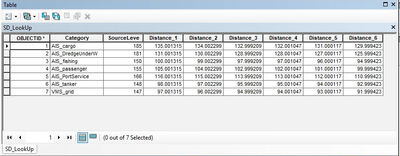# Inline model variables, Add Field calculations in ModelBuilder

351
3
05-27-2021 01:03 AMNew Contributor

As part of model I want to run, I have have a “SD_Lookup” table that is used as input data and contains the mean source level (dB) for 8 vessel categories and their corresponding dB levels of propagated noise for different buffer distances (picture 1 below).

I am trying to follow and repoduce what is presented in the Picture2 below.

In the instructions, it is indicated that the noise levels contained in this table should be saved as “model variables”. And that, when using the “Add Field” tool on a layer of a vessel category, a new field should be added with a name corresponding to the right source of buffer distance. This would be done thanks to calculations using the in-line variable ‘dB_%distance variable name%’. Finally the “Calculate field” tool should populate the fields with a value of 0.

My main issue is that I do not know how to save the noise levels as model variables and then I do not know what type of formula or calculation I should perform as ‘dB_%distance variable name%’ didn’t seem to work when I tried.

I can drag the SD_Lookup table in the model and transform it in a Model Parameter BUT, I cannot separate it by field like on the picture below (sourcelevel, distance_1, etc.). So I am wondering if what was done is that the same SD_Lookup table was dropped 7 times for each column and simply renamed. But then if this was the case, the formula later on in the calculate field “dB_%distance variable name%’ should work, although it doesn’t for me so far.

Could anyone detect where I made a mistake?Tags (3)
3 RepliesbyMVP Esteemed Contributor

There are two approaches to extracting values from a table of values into ModelBuilder variables that I know.

1. Create a table view and then use Select Layer By Attribute (or Iterate Row Selection if you want to dig into an iterator) to select each row, and then use the Get Field Value tool to get the data into model variables. This would probably be done best in a sub model, as we're talking about a lot of tools.

2. Use the Calculate Value tool to read the table in Python and do whatever you are doing in Python using Python variables, not ModelBuilder.  (This requires Python knowledge.)byMVP Esteemed Contributor

It may be help if you explain exactly what you are trying to do here. It looks like you are trying calculate table values back into a table. If that is what you are doing, why not just use the table?New Contributor

Thank you for your quick reply and your suggestions, although I don't think that I should be using Python, as it was not indicated in the report that I am following, made by a consultancy agency (can find the details here MMO Project No: 1097 - Modelled Mapping of Continuous Underwater Noise Generated by Activities – Tec...), my problem occures around page 27 of the report.

The picture above (page27) represents what I need to reproduce in the ModelBuilder, unfortunately, not many details are given. This part is a submodel for a the model page 25. From what I understood of the submodel, I need to use my reference table as a starting input point for future calculations.

The data called 'AIS_tanker' is a table composed of the density presence of vessels per cell grid. The SD-lookup table contains the average source level for each vessel category, including tankers. So by using the inline variable substitution, I thinkt that it will create a connection between these two tables, which will be used later on in the model to calculate the noise produced within each cell grid based on the average source of the vessel category and the density.

I don't know if this makes much sense, I'm still trying to get my head around it, especially because it is the first time I'm using the ModelBuilder tool.